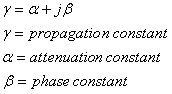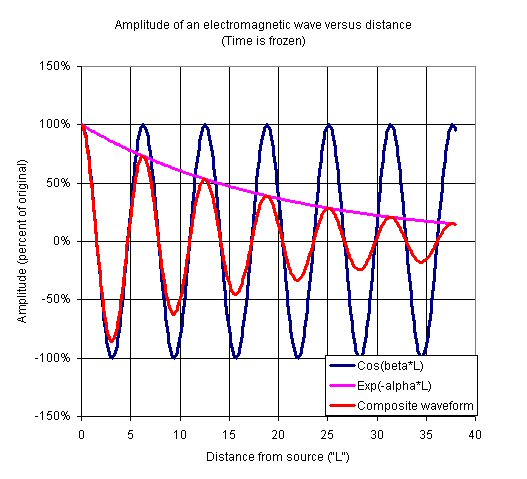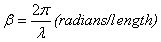# Propagation Constant

Click here to go to our page on wavenumber, which is often confused with propagation phase constant!

We placed the transmission line model onto its own page. The propagation constant is an important parameter associated with transmission lines. It is a complex number denoted by Greek lower case letter γ (gamma), and is used to describe the behavior of an electromagnetic wave along a transmission line.

### Propagation, attenuation and phase constants

The propagation constant is separated into two components that have very different effects on signals:The real part of the propagation constant is the attenuation constant and is denoted by Greek lowercase letter α (alpha). It causes a signal amplitude to decrease along a transmission line. The natural units of the attenuation constant are Nepers/meter, but we often convert to dB/meter in microwave engineering. To get loss in dB/length, multiply Nepers/length by 8.686. Note that attenuation constant is always a positive number, if it was negative you'd violate the First Law of Thermodymamics (you never get something for nothing!)

The phase constant is denoted by Greek lowercase letter β (beta) adds the imaginary component to the propagation constant. It determines the sinusoidal amplitude/phase of the signal along a transmission line, at a constant time. The phase constant's "natural" units are radians/meter, but we often convert to degrees/meter. A transmission line of length "l" will have an electrical phase of βl, in radians or degrees. To convert from radians to degrees, multiply by 180/π.

The two parts of the propagation constant have radically different effects on a wave. The amplitude of a wave (frozen in time) goes as cosine(βl). In a lossless transmission line, the wave would propagate as a perfect sine wave. In real life there is some loss to the transmission line, and that is where the attenuation constant comes in. The amplitude of the signal decays as Exp(-αl). The composite behavior of the propagation constant is observed when you multiply the effects of α and β.

The graph below represents wave propagation in a fairly lossy line, we made it lossy so you could observe the familiar exponential curve of amplitude decay. In this graph, β=1 and α=0.05. In practice we usually want to minimize loss, but this example is a very lossy line!### Phase constant versus wavenumber

We have a separate page on wavenumber. Phase constant and wavenumber are often treated as the same thing. Indeed, for TEM transmission lines (coax and stripline), the phase constant and wavenumber are equal. Waveguide is one case where you need to understand the difference between the two.

Wavenumber is denoted by lower case "k", and is a measure of how many cycles a wave has in a given length, for a traveling wave that is frozen in time.

### Phase constant, phase velocity, frequency and wavelength

Let's examine the relationships between phase constant, frequency, phase velocity and wavelength, Recall that there are 2π radians in a wavelength, therefore the relationship between phase constant and wavelength is simply:More to come!

### Transmission line model

This discussion of how the propagation constant can be derived from the transmission line model has been moved to a separate page.

We have a separate page on transmission line loss that deals further with the topic of splitting up the attenuation constant, check it out!

Author : Unknown Editor